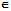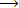Loading

# Describe the representation of complex numbers in algebra

Publish On: 2019-05-24

# jollay

Total Post: 559

## Question: Describe the representation of complex numbers in algebra

Reply On: 2013-10-08

# Mjay Jollay

Total Post: 0

## ANS: Describe the representation of complex numbers in algebra

Let CR be the set of complex numbers of the type (a, 0)

i.e. CR = {(a, 0) | aR}

Surely, CR ⊂ C. Now, it is possible to set up a one-one correspondence between the elements of CR and the elements of R, the set of real numbers.

Let ƒ : RCR be defined as

ƒ(a) = (a, 0),            ∀ aR

Let us now show that the mapping ƒ has a composition preserving property.

i.e. (i) ƒ(a + b) = (a + b, 0) = (a, 0) + (b, 0) = ƒ(a) + ƒ(b), ∀ a, bR

(ii) ƒ(ab) = (ab, 0) = (a, 0) (b, 0) = ƒ(a). ƒ(b), ∀ a, bR

Hence under the mapping ƒ, the addition and multiplication composition are preserved and also it associates to each real number R, whose first coordinate is a and the second coordinate is zero.

Let z = (a, b)C ; then

(a, b) = (a, 0) + (0, b)

= (a, 0) + (0, 1) (b, 0)                              (1)

Clearly, (0, 1) (0, 1) = (-1, 0) --1     [by the above form ƒ : CR  -R]

Let us denote (0, 1) by i; then

i2 = -1,

and from (1), we get

z = (a, b) = (a, 0) + (b, 0) = a + ib

Hence z = a + ib,

which is the usual notion of a complex number.

In this complex number, “a” is called the real part of complex number (a, b, and “b” is called the imaginary part of the complex number (a, b). A complex number is called purely real if its imaginary part is zero and it is called purely imaginary if its real part is zero but its imaginary part is not zero.

#### Submit Your Answer

warning: Please Login To Submit Your Answer
Like Us On Facebook for All Latest Updates